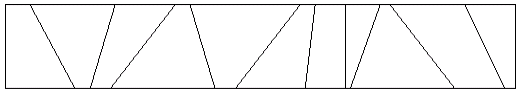# 2565. Toy Storage

Mom and dad have a problem: their child, Reza, never puts his toys away when he is finished playing with them. They gave Reza a rectangular box to put his toys in. Unfortunately, Reza is rebellious and obeys his parents by simply throwing his toys into the box. All the toys get mixed up, and it is impossible for Reza to find his favorite toys anymore.

Reza’s parents came up with the following idea. They put cardboard partitions into the box. Even if Reza keeps throwing his toys into the box, at least toys that get thrown into different partitions stay separate. The box looks like this from the top:We want for each positive integer $t$, such that there exists a partition with $t$ toys, determine how many partitions have $t$, toys.

### 输入格式

The input consists of a number of cases. The first line consists of six integers $n, m, x_1, y_1, x_2, y_2$. The number of cardboards to form the partitions is $n$ ($0 < n \le 1000$) and the number of toys is given in $m$ ($0 < m \le 1000$). The coordinates of the upper-left corner and the lower-right corner of the box are $(x_1, y_1)$ and $(x_2, y_2)$, respectively. The following $n$ lines each consists of two integers $U_i,L_i$, indicating that the ends of the $i$th cardboard is at the coordinates $(U_i, y_1)$ and $(L_i, y_2)$. You may assume that the cardboards do not intersect with each other. The next $m$ lines each consists of two integers $X_i, Y_i (x_1 \le X_i \le x_2, y_2 \le Y_i \le y_1)$ specifying where the $i$th toy has landed in the box. You may assume that no toy will land on a cardboard.

A line consisting of a single $0$ terminates the input.

### 输出格式

For each box, first provide a header stating Box on a line of its own. After that, there will be one line of output per count $(t > 0)$ of toys in a partition. The value $t$ will be followed by a colon and a space, followed the number of partitions containing $t$ toys. Output will be sorted in ascending order of $t$ for each box.

### 样例

Input
4 10 0 10 100 0
20 20
80 80
60 60
40 40
5 10
15 10
95 10
25 10
65 10
75 10
35 10
45 10
55 10
85 10
5 6 0 10 60 0
4 3
15 30
3 1
6 8
10 10
2 1
2 8
1 5
5 5
40 10
7 9
0

Output
Box
2: 5
Box
1: 4
2: 1


2 人解决，43 人已尝试。

2 份提交通过，共有 91 份提交。

9.9 EMB 奖励。